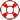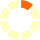Standard:

Description:

Standard:

Description:

Standard:

Description:

Standard:

Description:

Standard:

# Math.7.EE.4a or 7.EE.B.4.A

Description:

## Solve word problems leading to equations of the form px + q = r and p(x + q) = r, where p, q, and r are specific rational numbers. Solve equations of these forms fluently. Compare an algebraic solution to an arithmetic solution, identifying the sequence of the operations used in each approach. For example, the perimeter of a rectangle is 54 cm. Its length is 6 cm. What is its width?7th Grade Math - Linear Equations Lesson# Processing Request...